# Quadrature Amplitude Modulation (QAM): What is it?

Contents

## What is QAM (Quadrature Amplitude Modulation)?

QAM (Quadrature Amplitude Modulation) is defined as the modulation technique which is the combination of phase and amplitude modulation of a carrier wave into a single channel. In other words, QAM transmits information by changing both the amplitude and phase of a carrier wave, thereby doubling the effective bandwidth. QAM is also known as “quadrature carrier multiplexing”.

In a QAM signal, the direct modulation of a carrier wave in quadrature is involved. As a name “quadrature” indicates that the phase difference between two carriers is 90 degrees but each having the same frequency.

One signal is called the in-phase “I” signal, and the other is called the quadrature “Q” signal. Mathematically, one of the carrier signals can be represented by a sine wave (i.e.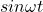) and the other can be represented by a cosine wave (i.e.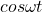).

The two modulated carrier signals are transmitted together at the source and at the destination, these two carrier signals are demodulated (i.e. separated) independently. To demodulate the signal coherent detection method is used.

The waveform of the QAM technique is shown below.

## Analog vs Digital QAM

### Analog QAM

The Analog QAMs are typically used to allow more than one signal to be carried on a single carrier. It is the same as the AM (Amplitude Modulation) with two carrier signals transmitted together of the same frequency but out of phase with 90 degrees.

Analog QAM is used in the transmission of chroma (color) information in PAL and NTSC analog video television systems. Where the I (in-phase) and Q (Quadrature) signals carry the components of chroma (color) information.

PAL stands for Phase Alternating Line is the video standard which is mostly used in the European and Asian countries and NTSC stands for National Television Standards Committee is the analog color television standard which is mostly used in South America and North America.

### Digital QAM

Digital QAMs are usually described as a “Quantized QAM” and they are commonly used in radio communications systems ranging from cellular technology to Wi-Fi. Digital QAM can carry higher data rates compared to both amplitude and phase-modulated scheme.

In digital QAM schemes, to define the values of phase and amplitude different points can be used. This is known as a constellation diagram. Thus a constellation diagram is the set of possible message points.

QAM can be realized by using a constellation diagram. In the constellation diagram, the constellation points are arranged in a square grid with equal horizontal and vertical distance. The minimum distance between the constellation points is known as a Euclidean distance.

In the digital communications, data is usually in a binary form and it has two states 0 or 1, so the number of constellation points in the grid is usually a power of 2 i.e. 2, 4, 8, 16, 32………… the most common formats of QAM are16-QAM (24), 32-QAM (25), 64-QAM (26), 128-QAM (27) and 256-QAM (28).

The bit sequence mapping for a 16-QAM is shown below in the constellation diagram. A diagram shows that binary values associated with different positions for a 16-QAM signal. It can be seen that a continuous bit stream may be represented as a sequence and divided into four groups in each of the four quadrants.

Normally a 16-QAM is considered as the lowest order QAM because 2-QAM is considered the same as for BPSK (i.e. Binary Phase Shift Keying) and 4-QAM is the same as QPSK (i.e. Quadrature Phase Shift Keying). In addition to the error-rate performance of 8-QAM is almost the same as that of 16-QAM hence it is not widely used.

## What Are QAM Channels?

QAM channels are the variety of different formats of QAMs which are used in many radio communications and data delivery applications. Some specific variants of QAMs channel are used in some specific applications and standards.

Let us assume that by using QAM we want to transmit a symbol consisting of 4 bits. That means N=4 and there are 24 = 16 different possible symbols. Hence the QAM system can generate 16 different distinguishable signals. It is known as a 16-QAM. By using 16-QAM we can modulate the carrier signal into any of 16 different phase and amplitude states.

Examples of some other QAM formats or channels are 16-QAM, 32-QAM, 64-QAM,128-QAM, and 256-QAM.

The number of QAM symbols or states is determined by the number of binary bits per second.

The number of possible symbols for QAM channels is given byHere, the term “symbol” means a combination of amplitude and phase.

Depending on the number of bits per message or symbol the QAM signals are classified as follows:

### 16 QAM

16 QAM is one of the different formats of QAM in which the carrier signal is modulated into any of 16 different phase and amplitude states.

#### How many bits per symbol can be transmitted using 16-QAM?

By using 16-QAM 4 bits per symbol can be transmitted.

### 32 QAM

32 QAM is one of the different formats of QAM in which the carrier signal is modulated into any of 32 different phase and amplitude states.

#### How many bits per symbol can be transmitted using 32-QAM?

By using 32-QAM 5 bits per symbol can be transmitted.

### 64 QAM

64 QAM is one of the different formats of QAM in which the carrier signal is modulated into any of 64 different phase and amplitude states.

#### How many bits per symbol can be transmitted using 64-QAM?

By using 64-QAM 6 bits per symbol can be transmitted.

### 128 QAM

128 QAM is one of the different formats of QAM in which the carrier signal is modulated into any of 128 different phase and amplitude states.

#### How many bits per symbol can be transmitted using 128-QAM?

By using 128-QAM 7 bits per symbol can be transmitted.

### 256 QAM

256 QAM is one of the different formats of QAM in which the carrier signal is modulated into any of 256 different phase and amplitude states.

#### How many bits per symbol can be transmitted using 256-QAM?

By using 256-QAM 8 bits per symbol can be transmitted.

### Bandwidth of QAM System

The plot of power spectral density for the QAM system is shown in the below figure.

The main portion of the frequency spectrum extends from –fs to +fs. Therefore the bandwidth of QAM system is given by,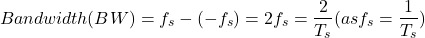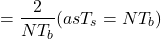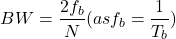## QAM vs QPSK vs BPSK vs OOK

The comparison of various modulation techniques has been discussed in the table below.

Note that a Euclidean distance is a distance between two points in the constellation diagram.

QAM can transmit the data at four times the bit rate over the same bandwidth as BPSK. Therefore, QAM is considered to be a bandwidth-efficient modulation technique.

Some of the advantages of QAM include:

• Noise immunity of QAM’s is very high hence noise interference is very less.
• QAM has a low probability of error value.
• QAM supports a high data rate. So that the number of bits can be carried by the carrier signal. Hence, it is mostly used in wireless communication systems.
• QAM has a doubling the effective bandwidth.
• By using both sine wave and cosine wave into single-channel the communication channel capacity is doubled compared to the use of only one sine wave or one cosine wave.

Some of the disadvantages of QAM include:

• In QAM, amplitude changes are susceptible to noise.
• It is not necessary to use of linear amplifier in a radio transmitter when a phase or frequency modulated signal is amplified, but due to the presence of amplitude component in QAM, it is necessary to use the linear amplifier in order to maintain linearity. These linear amplifiers are less efficient and consume more power.
• It is possible to transmit more bits per symbol but in higher-order QAM formats the constellation points are closely spaced which is more susceptible to noise and produces errors in the data.
• Also in higher-order QAM formats, there is a difficulty for the receiver to decode the signal appropriately. In other words, there is reduced noise immunity. So the higher-order QAM formats are only used when there is a high signal to noise ratio.

## Applications of QAM

Some of the applications of QAM include:

• QAM technique is widely used in the radio communications field because of the increase of the bit data rate.
• QAM is used in applications ranging from short-range wireless communications to long-distance telephone systems.
• QAM is used in microwave and telecommunication systems to transmit the information.
• The 64 QAM and 256 QAM are used in digital cable television and cable modem.
• QAM is used in optical fiber systems to increase bit rates.
• It is used in many communication systems like Wi-Fi, Digital Video Broadcast (DVB), and WiMAX.
Want To Learn Faster? 🎓
Get electrical articles delivered to your inbox every week.
No credit card required—it’s 100% free.